# What happens with a non-unitary production amount in LCA?Figure 1: One snail on a stick.

## Production amounts in linear LCA

LCA is normally performed using a set of linear equations that describe the inputs and outputs of the world economy, and their resulting biosphere emissions and resource consumption. We typically have a technosphere matrix like this: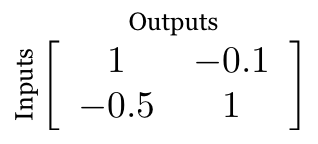This formulation is not as precise as it could be, however. It makes a very common mistake in matrix LCA - it conflates processes and products (flows). Here are more accurate matrix labels: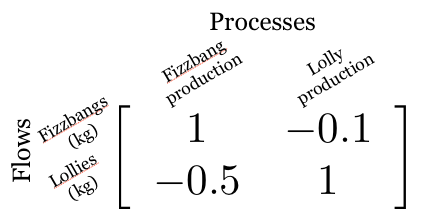Note that the rows are objects with physical units, like mass or energy, while the columns are the process of making or doing something, and as such are unitless.

Note

See also this blog post on multi-output processes in LCA (coming soon)

## Supply and demand

In this matrix, the process "Fizzbang production" produces 1 kilogram of fizzbangs (positive number), and consumes 0.5 kilograms of input lollies. Similarly, the process "Lolly production" produces 1 kilogram of lollies, and consumes 0.1 kilograms of input fizzbangs. If our final demand (functional unit) is one kilogram of fizzbang, then we need to solve the linear system: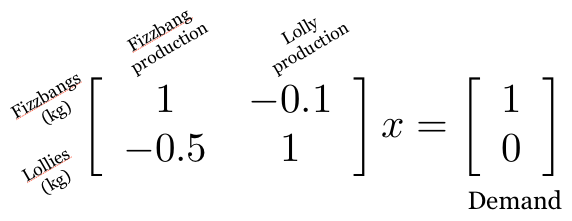Here, the vector x is the supply vector - the amount of each process required to produce the final demand. Let's check the units: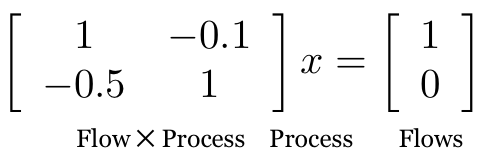And here we reach a critical point in understanding the mathematics of LCA: the demand vector has units of products (flows), while the supply vector has "units" of the amount needed of each process.

Let's quickly solve this linear system:

import numpy as np
a = np.array(((1, -0.1), (-0.5, 1)))
demand = np.array((1, 0))
np.linalg.solve(a, demand)
>>> array([ 1.05263158,  0.52631579])


To make sure we understand each other, the solution to this set of linear equations is telling us that to satisfy the final demand of 1 kilogram of fizzbang, we need 1.05 units of the process "Fizzbang production", and 0.53 units of the process "Lolly production".

## Non-unitary production

What happens when our processes don't produce one unit of the final product? After all, the choice of one unit of output is arbitrary - each process is a collection of input and output flows, and as such can scaled up or down. Let's see what happens when we scale the process "Fizzbang production" up by a factor of two: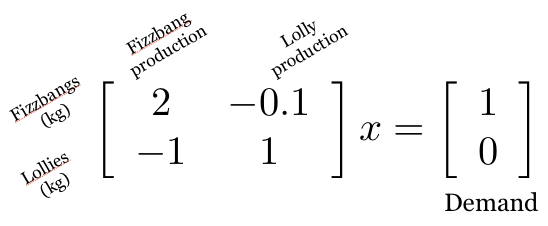a = np.array(((2, -0.1), (-1, 1)))
np.linalg.solve(a, demand)
>>> array([ 0.52631579,  0.52631579])


For this system, the amount of "Lolly production" remains, the same, but the amount of "Fizzbang production" is now halved, to 0.53.

This is the second critical point: non-unitary production values make it less easy to understand the meaning of the supply vector, because one unit of supply no longer corresponds to one unit of final output. Therefore, in my opinion, non-unitary production values, while mathematically possible, are not recommended, as they can make LCA results more confusing.

So $A^{-1}f$ has "units" of processes, and $B$ has units of physical biosphere flows per process. This means that biosphere flows can scale up and down along with the rest of the process inputs and outputs, but the life cycle inventory result will be the same regardless of the chosen production amount.